# homomorphism

## Sidorenko's Conjecture ★★★

Author(s): Sidorenko

Conjecture   For any bipartite graphand graph, the number of homomorphisms fromtois at least.

## Algorithm for graph homomorphisms ★★

Author(s): Fomin; Heggernes; Kratsch

Question

Is there an algorithm that decides, for input graphsand, whether there exists a homomorphism fromtoin timefor some constant?

## Hedetniemi's Conjecture ★★★

Author(s): Hedetniemi

Conjecture   Ifare simple finite graphs, then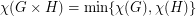.

Here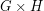is the tensor product (also called the direct or categorical product) ofand.

## Weak pentagon problem ★★

Author(s): Samal

Conjecture   Ifis a cubic graph not containing a triangle, then it is possible to color the edges ofby five colors, so that the complement of every color class is a bipartite graph.

## Mapping planar graphs to odd cycles ★★★

Author(s): Jaeger

Conjecture   Every planar graph of girth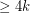has a homomorphism to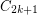.

Keywords: girth; homomorphism; planar graph

## Pentagon problem ★★★

Author(s): Nesetril

Question   Letbe a 3-regular graph that contains no cycle of length shorter than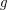. Is it true that for large enough~there is a homomorphism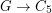?

Keywords: cubic; homomorphism

## A homomorphism problem for flows ★★

Author(s): DeVos

Conjecture   Letbe abelian groups and let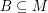andsatisfyand. If there is a homomorphism from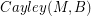to, then every graph with a B-flow has a B'-flow.

Keywords: homomorphism; nowhere-zero flow; tension